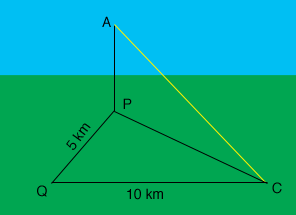SEARCH HOMEMath Central Quandaries & QueriesQuestion from Varun, a student: An aircraft is vertically above a point which is 10 kms west and 5 kms north of a control tower. if the aircraft is 4000 mts above the ground,how far is it from the control tower?Hi,

Here is my diagram of the situation you describe.7

The control tower is at C, the aircraft is at A, P is on the ground directly below the aircraft and Q is 10 km west of C and 5 km south of P.

The angle QC is a right angle and hence you can use Pythagoras' theorem to find the distance from P to C. The angle APC is a right angle also so you can use Pythagoras' theorem again, this tim on triangle APC.

I hope this helps,
PennyMath Central is supported by the University of Regina and The Pacific Institute for the Mathematical Sciences.